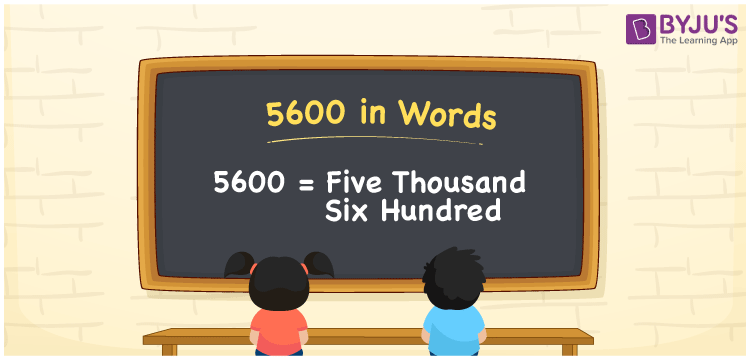# 5600 in Words

We can write 5600 in words as Five thousand six hundred. Suppose your teacher wrote 5600 on a board, then you may read that number as “Five thousand six hundred. In this way, we can pronounce cardinal numbers in words. In this article, you will get the conversion of 5600 to word form with the help of a place value chart.

 5600 in words Five thousand six hundred Five thousand six hundred in Numbers 5600

## 5600 in English Words

We generally write numbers in words with the help of the English alphabet. Thus, we can express 5600 in English words as “Five thousand six hundred”.## How to Write 5600 in Words?

Let us make the place value chart for 5600 with four columns as it has four digits. The below table shows the place value of each of the four digits of the number 5600.

 Thousands Hundreds Tens Ones 5 6 0 0

Here, ones = 0, tens = 0, hundreds = 6, and thousands = 5.

So, 5 × Thousand + 6 × Hundred + 0 × Ten + 0 × One

= 5 × 1000 + 6 × 100 + 0 × 10 + 0 × 1

= 5000 + 600

= Five thousand + Six hundred

= Five thousand six hundred

Therefore, 5600 in words = Five thousand six hundred.

Also, check: place value

5600 is a natural number that precedes 5601 and succeeds 5599.

5600 in words – Five thousand six hundred

Is 5600 an odd number? – No

Is 5600 an even number? – Yes

Is 5600 a prime number? – No

Is 5600 a composite number? – Yes

Is 5600 a perfect square number? – No

Is 5600 a perfect cube number? – No

## Frequently Asked Questions on 5600 in Words

Q1

### How do you write 5600 in words?

The number 5600 can be written in words as Five thousand six hundred.
Q2

### How to write Rs. 5600 in words on a cheque?

On a cheque, Rs. 5600 will be written in words as Five thousand six hundred rupees only.
Q3

### Express the value of 3400 + 2200 in words.

3400 + 2200 = 5600 The value of 3400 + 2200 in words is five thousand and six hundred.